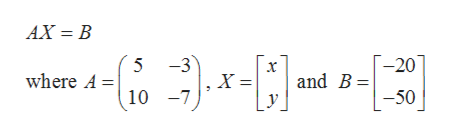# For Exercise, solve the system by using the inverse of the coefficient matrix.5x-3y=-2010x-7y=-50

Question
1 views

For Exercise, solve the system by using the inverse of the coefficient matrix.

5x-3y=-20

10x-7y=-50

check_circle

Step 1

Given,

Step 2

The given system can be written in matrix form ashelp_outlineImage TranscriptioncloseAX = B -20 and B= -3 5 where A = 10 -7 -50 , fullscreen
Step 3

Now

...

### Want to see the full answer?

See Solution

#### Want to see this answer and more?

Solutions are written by subject experts who are available 24/7. Questions are typically answered within 1 hour.*

See Solution
*Response times may vary by subject and question.
Tagged in

### Matrices